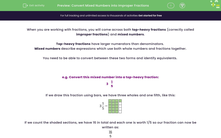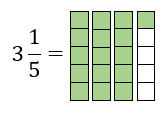# Convert Mixed Numbers into Improper Fractions

In this worksheet, students will practise converting mixed numbers (whole numbers and fractions) into top-heavy (improper) fractions. This is a very useful skill to use when adding or subtracting fractions.Key stage:  KS 4

Year:  GCSE

GCSE Subjects:   Maths

GCSE Boards:   Pearson Edexcel, OCR, Eduqas, AQA,

Curriculum topic:   Number, Fractions, Decimals and Percentages

Curriculum subtopic:   Structure and Calculation Fractions

Difficulty level:#### Worksheet Overview

When you are working with fractions, you will come across both top-heavy fractions (correctly called improper fractions) and mixed numbers.

Top-heavy fractions have larger numerators than denominators.

Mixed numbers describe expressions which use both whole numbers and fractions together.

You need to be able to convert between these two forms and identify equivalents.

e.g. Convert this mixed number into a top-heavy fraction:

3
 1 5

If we draw this fraction using bars, we have three wholes and one fifth, like this:If we count the shaded sections, we have 16 in total and each one is worth 1/5 so our fraction can now be written as:

 16 5

Doing this the quicker way...

You should have noticed that the denominator is the same in both the question and answer fractions. This is ALWAYS true.

So we just need to find the numerator.

If we have 3 wholes which we are splitting into fifths, we have 3 x 5 = 15 fifths.

We need to remember that we had an extra 1 fifth in the question too, which gives us 16 overall.

To summarise:

In order to find the new numerator, multiply the whole number by the denominator then add the numerator.

The denominator will stay the same in both fractions.

In this activity, you will convert mixed numbers (so whole numbers and fractions) into top-heavy, improper fractions.

This is a very useful skill to use when adding or subtracting fractions.

### What is EdPlace?

We're your National Curriculum aligned online education content provider helping each child succeed in English, maths and science from year 1 to GCSE. With an EdPlace account you’ll be able to track and measure progress, helping each child achieve their best. We build confidence and attainment by personalising each child’s learning at a level that suits them.

Get started